## [問題]如何修改(隱藏)註冊時不需填入的欄位

tifeng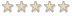### [問題]如何修改(隱藏)註冊時不需填入的欄位

Mac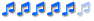~Mac
+ 關於 phpBB 使用問題請在版面發問，私人訊息提供其他不相干或是隱私的事情聯絡之用。

phpBB 官網 | 竹貓星球 | MacphpBBMOD | 我的服務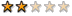NOFX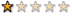<!-- BEGIN switch_user_logged_in -->

<!-- END switch_user_logged_in -->

trying!!!..........................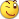54yuwei### Re: [問題]修改註冊的欄位

tifeng 寫:請問一下

``````<input type="radio" name="viewemail" value="1" {VIEW_EMAIL_YES} />
<span class="gen">{L_YES}</span>
``````

54yuweiICQ 號碼欄位，請尋找下面原始碼

``````<tr>
<td class="row1"><span class="gen">{L_ICQ_NUMBER}:</span></td>
<td class="row2">
<input type="text" name="icq" class="post"style="width: 100px"  size="10" maxlength="15" value="{ICQ}" />
</td>
</tr>
``````

``````<tr>
<td class="row1"><span class="gen">{L_AIM}:</span></td>
<td class="row2">
<input type="text" class="post"style="width: 150px"  name="aim" size="20" maxlength="255" value="{AIM}" />
</td>
</tr>
``````
MSN Messenger欄位，請尋找下面原始碼

``````<tr>
<td class="row1"><span class="gen">{L_MESSENGER}:</span></td>
<td class="row2">
<input type="text" class="post"style="width: 150px"  name="msn" size="20" maxlength="255" value="{MSN}" />
</td>
</tr>
``````

``````<tr>
<td class="row1"><span class="gen">{L_YAHOO}:</span></td>
<td class="row2">
<input type="text" class="post"style="width: 150px"  name="yim" size="20" maxlength="255" value="{YIM}" />
</td>
</tr>
``````

``````	<tr>
<td class="row1"><span class="gen">{L_WEBSITE}:</span></td>
<td class="row2">
<input type="text" class="post"style="width: 200px"  name="website" size="25" maxlength="255" value="{WEBSITE}" />
</td>
</tr>

``````

``````<tr>
<td class="row1"><span class="gen">{L_LOCATION}:</span></td>
<td class="row2">
<input type="text" class="post"style="width: 200px"  name="location" size="25" maxlength="100" value="{LOCATION}" />
</td>
</tr>
``````